# Net Profit Margin

Net income divided by total revenue, expressed as a percentage

## What is Net Profit Margin?

Net Profit Margin (also known as “Profit Margin” or “Net Profit Margin Ratio”) is a financial ratio used to calculate the percentage of profit a company produces from its total revenue. It measures the amount of net profit a company obtains per dollar of revenue gained. The net profit margin is equal to net profit (also known as net income) divided by total revenue, expressed as a percentage.The typical profit margin ratio of each company can be different depending on which industry the company is in. As a financial analyst, it’s important in day-to-day financial analysis.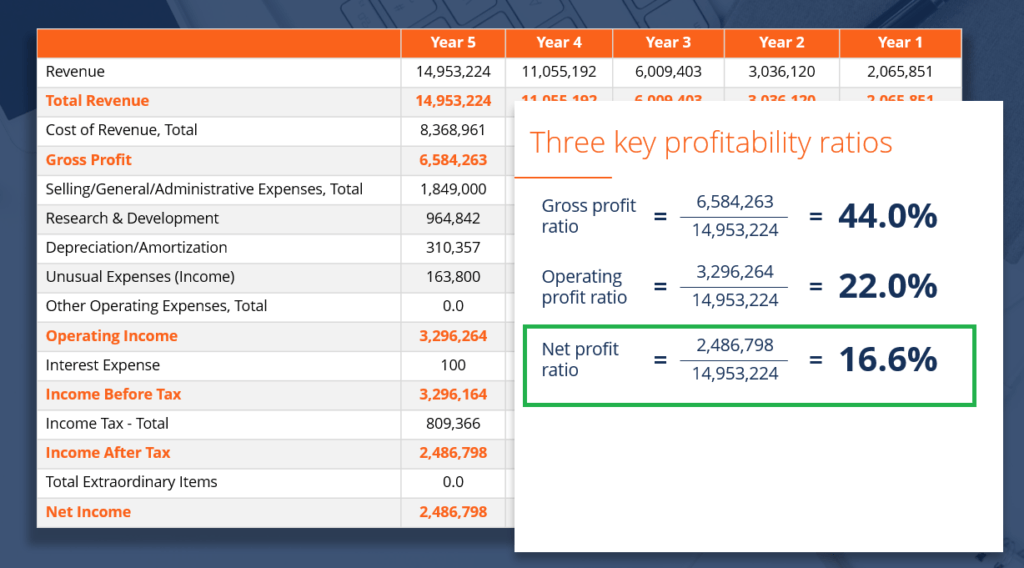Source: CFI Financial Analysis Fundamentals Course.

### Net Profit Margin Formula

Net Profit margin = Net Profit  ⁄  Total revenue x 100

Net profit is calculated by deducting all company expenses from its total revenue. The result of the profit margin calculation is a percentage – for example, a 10% profit margin means for each \$1 of revenue the company earns \$0.10 in net profit. Revenue represents the total sales of the company in a period.

#### Calculation Example #1

Company XYZ and ABC both operate in the same industry. Which company has a higher net profit margin?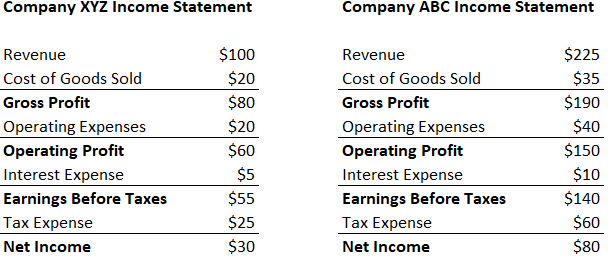Step 1: Write out the formula

Net Profit Margin = Net Profit/Revenue

Step 2: Calculate the net profit margin for each company

Company XYZ:

Net Profit Margin = Net Profit/Revenue = \$30/\$100 = 30%

Company ABC:

Net Profit Margin = Net Profit/Revenue = \$80/\$225 = 35.56%

Company ABC has a higher net profit margin.

#### Calculation Example #2

Company A and company B have net profit margins of 12% and 15% respectively. Both companies earned \$150 in revenue. How much net profit did each company make?

Step 1: Write out formula

Net Profit Margin = Net Profit/Revenue

Net Profit = Net Margin * Revenue

Step 2: Calculate net profit for each company

Company A:

Net Profit = Net Margin * Revenue = 12% * \$150 = \$18

Company B:

Net Profit = Net Margin * Revenue = 15% * \$150 = \$22.50

#### Calculation Example #3

Company A and B earned \$83.50 and \$67.22 in net profit respectively. Both companies have a net profit margin of 18.22%. How much revenue did each company earn?

Step 1: Write out formula

Net Profit Margin = Net Profit/Revenue

Revenue = Net Profit/Net Profit Margin

Step 2: Calculate revenue for each company

Company A:

Revenue = \$83.50/18.22% = \$458.29

Company B:

Revenue = \$67.22/18.22% = \$368.94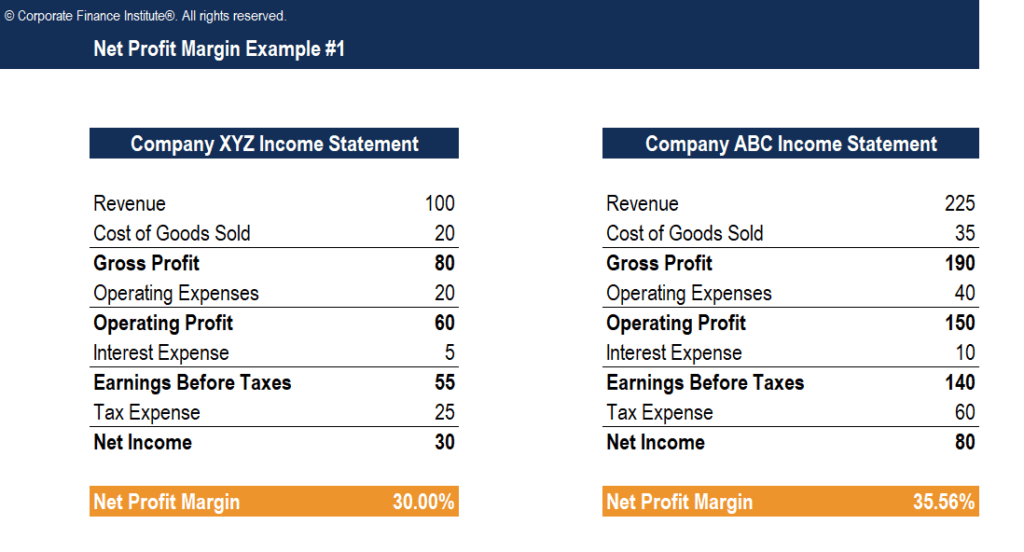### Video Explanation of Net Profit margin

Below is a video explanation from CFI’s Financial Analysis Fundamentals Course of how net profit margin is calculated and what it means when analyzing a company’s performance.

Get more video tutorials in CFI’s Financial Analyst Training Program.

### Understanding the Ratio

The net profit margin ratio is used to describe a company’s ability to produce profit and to consider several scenarios, such as an increase in expenses which is deemed ineffective. It is used extensively in financial modeling and company valuation.

Net profit margin is a strong indicator of a firm’s overall success and is usually stated as a percentage. However, keep in mind that a single number in a company report is rarely adequate to point out overall company performance. An increase in revenue might translate to a loss if followed by an increase in expenses. On the other hand, a decrease in revenue, followed by tight control over expenses, might put the company further in profit.

Other common financial metrics are EBITDA and Gross Profit.

A high net profit margin means that a company is able to effectively control its costs and/or provide goods or services at a price significantly higher than its costs. Therefore, a high ratio can result from:

• Efficient management
• Low costs (expenses)
• Strong pricing strategies

A low net profit margin means that a company uses an ineffective cost structure and/or poor pricing strategies. Therefore, a low ratio can result from:

• Inefficient management
• High costs (expenses)
• Weak pricing strategies

Investors need to take numbers from the profit margin ratio as an overall image of company profitability performance and initiate deeper research on the cause of an increase or decrease in the profitability as needed.

### Limitations of Net Profit Margin Ratio

When calculating the net profit margin ratio, analysts commonly compare the figure to different companies to determine which business performs the best.

While this is common practice, the net profit margin ratio can greatly differ between companies in different industries. For example, companies in the automotive industry may report a high profit margin ratio but lower revenue as compared to a company in the food industry.  A company in the food industry may show a lower profit margin ratio, but higher revenue.

It is recommended to compare only companies in the same sector with similar business models.

Other limitations include the possibility of misinterpreting the profit margin ratio and cash flow figures. A low net profit margin does not always indicate a poorly performing company. Also, a high net profit margin does not necessarily translate to high cash flows.

#### Limitations Example #1 – Comparing Companies

A jewelry company that sells a few expensive products may have a much higher profit margin as compared to a grocery store that sells many cheap products.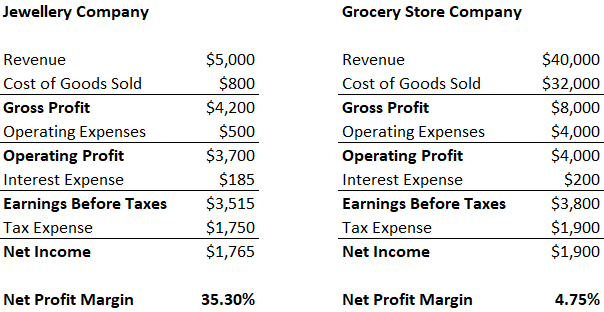It’d be inappropriate to compare the margins for these two companies, as their operations are completely different.

#### Limitations Example #2 – Companies with Debt

If a company has higher financial leverage than another, the firm with more debt financing may have a smaller net profit margin due to the higher interest expenses. This negatively affects net profit, lowering the net profit margin for the company.

#### Limitations Example #3 – Depreciation Expense

Companies with high property plant & equipment (PP&E) assets will be affected by higher depreciation expenses, lowering the firm’s net profit margin. This may be misleading because the company could have significant cash flow but may seem inferior due to their lower profit margin.

#### Limitations Example #4 – Manipulation of Profit

Management may reduce long-term expenses (such as research and development) to increase their profit in the short-term. This can mislead investors looking at net margin, as a company can boost their margin temporarily.

### Financial Analysis

Calculating the net margin of a business is a routine part of financial analysis. It is part of a type of analysis known as vertical analysis, which takes every line item on the income statement and divides it into revenue. To compare the margin for a company on a year-over-year (YoY) basis, a horizontal analysis is performed. To learn more, read CFI’s free guide to analyzing financial statements.

To learn more via online courses, check out our wide ranges of topics such as: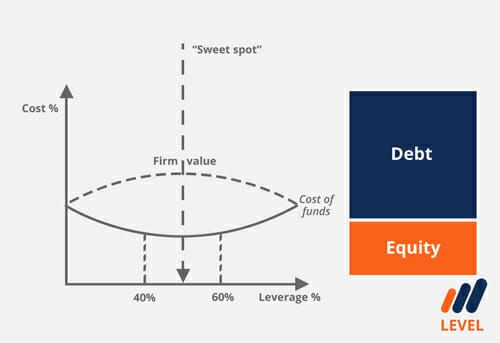Thank you for reading our guide to the net margin formula. CFI is the official global provider of the Financial Modeling and Valuation Analyst (FMVA)™ certification, designed to transform anyone into a world-class financial analyst.

• Gross Profit Margin
• EBITDA Margin
• Free Cash Flow Guide
• Financial Modeling Guide

### Financial Analyst Training

Get world-class financial training with CFI’s online certified financial analyst training program!

Gain the confidence you need to move up the ladder in a high powered corporate finance career path.

Learn financial modeling and valuation in Excel the easy way, with step-by-step training.